Question

Determine the effective spring constant of the suspension system of a car. Consider a load of 4 passengers, each with a mass of 70 kg. The car suspension system consists of four identical springs, each with a spring constant k. When all four people get into the car, the tires are depressed by about Δx = 2.0 cm.

1.haidang

The value of spring constant for each spring of the suspension system of a car   K = 343.35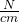Explanation:

Total force on the springs = weight of the four passengers

⇒ F = 4 × 70 × 9.81

⇒ F = 2746.8 N

In the suspension system of the car the four springs are connected in parallel. So Equivalent spring constant is given by,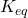= 4 K ——– ( 1 )

Depression in the spring Δx = 2 cm

Now the force  on the spring is given by

F =× Δx=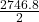= 1373.4Now the spring constant for each spring =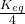⇒ K =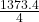⇒ K = 343.35This is the value of spring constant for each spring of the suspension system of a car.